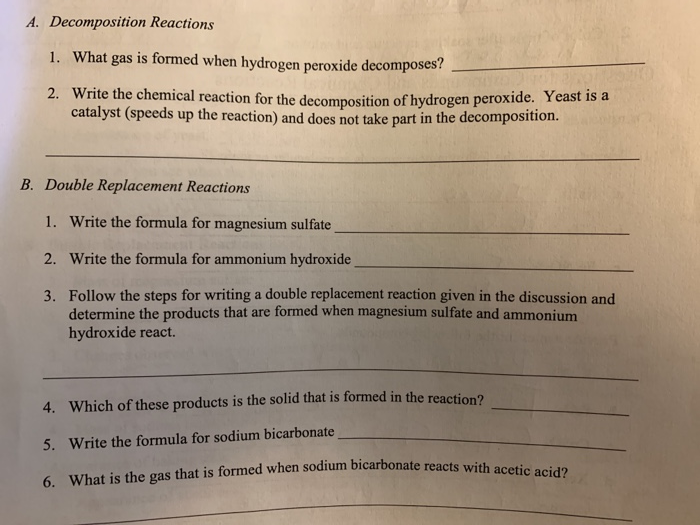# Solved: A. Decomposition Reactions 1. What Gas Is Formed When Hydrogen Peroxide Decomposes? Write The Chemical Reaction For The Decomposition Of Hydrogen Peroxide. Yeast Is A Catalyst (speeds Up The R

By |A. Decomposition Reactions 1. What gas is formed when hydrogen peroxide decomposes? Write the chemical reaction for the decomposition of hydrogen peroxide. Yeast is a catalyst (speeds up the reaction) and does not take part in the decomposition. 2. Double Rep lacement Reactions B. 1. Write the formula for magnesium sulfate 2. Write the formula for ammonium hydroxide Follow the steps for writing a double replacement reaction given in the discussion and determine the products that are formed when magnesium sulfate and ammonium hydroxide react. 3. 4. Which of these products is the solid that is formed in the reaction? 5. Write the formula for sodium bicarbonate 6. What is the gas that is formed when sodium bicarbonate reacts with acetic acid?